# 8.7: 求解激进方程

•• OpenStax
• OpenStax
$$\newcommand{\vecs}{\overset { \rightharpoonup} {\mathbf{#1}} }$$ $$\newcommand{\vecd}{\overset{-\!-\!\rightharpoonup}{\vphantom{a}\smash {#1}}}$$$$\newcommand{\id}{\mathrm{id}}$$ $$\newcommand{\Span}{\mathrm{span}}$$ $$\newcommand{\kernel}{\mathrm{null}\,}$$ $$\newcommand{\range}{\mathrm{range}\,}$$ $$\newcommand{\RealPart}{\mathrm{Re}}$$ $$\newcommand{\ImaginaryPart}{\mathrm{Im}}$$ $$\newcommand{\Argument}{\mathrm{Arg}}$$ $$\newcommand{\norm}{\| #1 \|}$$ $$\newcommand{\inner}{\langle #1, #2 \rangle}$$ $$\newcommand{\Span}{\mathrm{span}}$$ $$\newcommand{\id}{\mathrm{id}}$$ $$\newcommand{\Span}{\mathrm{span}}$$ $$\newcommand{\kernel}{\mathrm{null}\,}$$ $$\newcommand{\range}{\mathrm{range}\,}$$ $$\newcommand{\RealPart}{\mathrm{Re}}$$ $$\newcommand{\ImaginaryPart}{\mathrm{Im}}$$ $$\newcommand{\Argument}{\mathrm{Arg}}$$ $$\newcommand{\norm}{\| #1 \|}$$ $$\newcommand{\inner}{\langle #1, #2 \rangle}$$ $$\newcommand{\Span}{\mathrm{span}}$$$$\newcommand{\AA}{\unicode[.8,0]{x212B}}$$

##### 学习目标

• 求解激进方程
• 用两个自由基求解激进方程
• 在应用中使用自由基

1. 简化：$$(y−3)^{2}$$
如果您错过了此问题，请查看示例 5.31。
2. 解决：$$2x−5=0$$
如果您错过了此问题，请查看示例 2.2。
3. 解决$$n^{2}−6n+8=0$$
如果您错过了此问题，请查看示例 6.45。

## 求解激进方程

##### 示例$$\PageIndex{1}$$ how to solve a radical equation

 步骤 1：分离方程一侧的自由基。 要隔离激进分子，$$9$$请在两边加入。 简化。 $$\begin{array}{c}{\sqrt{5 n-4}-9=0} \\ {\sqrt{5 n-4}-9\color{red}{+9}\color{black}{=}0\color{red}{+9}} \\ {\sqrt{5 n-4}=9}\end{array}$$ 步骤 2：将方程的两边提高到指数的乘方。 由于平方根的索引为$$2$$，因此我们将两边进行平方。 $$(\sqrt{5 n-4})^{2}=(9)^{2}$$ 步骤 3：求解新方程。 记住，$$(\sqrt{a})^{2}=a$$。 \begin{aligned} 5 n-4 &=81 \\ 5 n &=85 \\ n &=17 \end{aligned} 第 4 步：检查原始方程中的答案。 检查答案。 $$\begin{array}{r}{\sqrt{5 n-4}-9=0} \\ {\sqrt{5(\color{red}{17}\color{black}{)}-4}-9 \stackrel{?}{=} 0} \\ {\sqrt{85-4}-9 \stackrel{?}{=} 0} \\ {\sqrt{81}-9 \stackrel{?}{=} 0} \\ {9-9=0} \\ {0=0}\end{array}$$ 解决办法是$$n=17$$。
##### 练习$$\PageIndex{1}$$

$$m=\frac{23}{3}$$

##### 练习$$\PageIndex{2}$$

$$z=\frac{3}{10}$$

### 用一个自由基求解一个激进方程

1. 分离方程一侧的自由基。
2. 将方程的两边提高到指数的幂次方。
3. 求解新方程。
4. 检查原始方程式中的答案。

##### 示例$$\PageIndex{2}$$要隔离激进分子，$$1$$请将两边减去。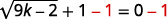简化。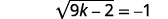##### 练习$$\PageIndex{4}$$

$$\begin{array}{l}{(a+b)^{2}=a^{2}+2 a b+b^{2}} \\ {(a-b)^{2}=a^{2}-2 a b+b^{2}}\end{array}$$

##### 示例$$\PageIndex{3}$$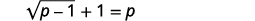要隔离激进分子，请$$1$$从两边减去。简化。对方程的两边进行平方。使用右侧的二项式正方形乘积图案进行简化，然后求解新方程。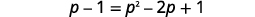它是一个二次方程，所以在一边求零。将右侧考虑在内。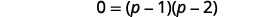使用 “零积分” 属性。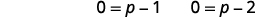求解每个方程。查看答案。##### 练习$$\PageIndex{5}$$

$$x=2, x=3$$

##### 练习$$\PageIndex{6}$$

$$y=5, y=6$$

$$(\sqrt{a})^{3}=a$$

##### 示例$$\PageIndex{4}$$

 $$\sqrt{5 x+1}+8=4$$ 要隔离激进分子，请$$8$$从两边减去。 $$\sqrt{5 x+1}=-4$$ 将方程的两边立方块。 $$(\sqrt{5 x+1})^{3}=(-4)^{3}$$ 简化。 $$5 x+1=-64$$ 求解方程。 $$5 x=-65$$ $$x=-13$$ 检查答案。解决办法是$$x=-13$$。
##### 练习$$\PageIndex{7}$$

$$x=-6$$

##### 练习$$\PageIndex{8}$$

$$x=-9$$

$$\left(x^{\frac{1}{2}}\right)^{2}=x,\left(x^{\frac{1}{3}}\right)^{3}=x$$

##### 示例$$\PageIndex{5}$$

 $$(3 x-2)^{\frac{1}{4}}+3=5$$ 要用有理指数分离该项，请$$3$$从两边减去。 $$(3 x-2)^{\frac{1}{4}}=2$$ 将方程的每一边提高到第四次方。 $$\left((3 x-2)^{\frac{1}{4}}\right)^{4}=(2)^{4}$$ 简化。 $$3 x-2=16$$ 求解方程。 $$3x=18$$ $$x=6$$ 检查答案。解决办法是$$x=6$$。
##### 练习$$\PageIndex{9}$$

$$x=8$$

##### 练习$$\PageIndex{10}$$

$$x=6$$

##### 示例$$\PageIndex{6}$$

 $$\sqrt{r+4}-r+2=0$$ 隔离激进分子。 $$\sqrt{r+4}=r-2$$ 对方程的两边进行平方。 $$(\sqrt{r+4})^{2}=(r-2)^{2}$$ 简化然后求解方程。 $$r+4=r^{2}-4 r+4$$ 如果是二次方程，则在一侧取零。 $$0=r^{2}-5 r$$ 将右侧考虑在内。 $$0=r(r-5)$$ 使用 “零积分” 属性。 $$0=r \quad 0=r-5$$ 求解方程。 $$r=0 \quad r=5$$ 检查你的答案。解决办法是$$r=5$$。 $$r=0$$是一个极端的解决方案。
##### 练习$$\PageIndex{11}$$

$$m=7$$

##### 练习$$\PageIndex{12}$$

$$n=3$$

##### 示例$$\PageIndex{7}$$

 $$3 \sqrt{3 x-5}-8=4$$ 分离激进术语。 $$3 \sqrt{3 x-5}=12$$ 通过将两边除以来隔离激进分子$$3$$。 $$\sqrt{3 x-5}=4$$ 对方程的两边进行平方。 $$(\sqrt{3 x-5})^{2}=(4)^{2}$$ 简化，然后求解新方程。 $$3 x-5=16$$ $$3x=21$$ 求解方程。 $$x=7$$ 检查答案。解决办法是$$x=7$$。
##### 练习$$\PageIndex{13}$$

$$a=63$$

##### 练习$$\PageIndex{14}$$

$$b=311$$

## 用两个自由基求解激进方程

##### 示例$$\PageIndex{8}$$

$$\sqrt{4 x-3}=\sqrt{3 x+2}$$

$$(\sqrt{4 x-3})^{3}=(\sqrt{3 x+2})^{3}$$

\begin{aligned} 4 x-3 &=3 x+2 \\ x-3 &=2 \\ x &=5 \end{aligned}

##### 练习$$\PageIndex{15}$$

$$x=3$$

##### 练习$$\PageIndex{16}$$

$$x=-\frac{6}{5}$$

##### 示例$$\PageIndex{9}$$ how to solve a radical equation

 步骤 1：在方程的一侧分离出其中一个基项。 右翼的激进分子是孤立的。 $$\sqrt{m}+1=\sqrt{m+9}$$ 步骤 2：将方程的两边提高到指数的乘方。 我们把两边都弄平。 简化——乘法时要非常小心！ $$(\sqrt{m}+1)^{2}=(\sqrt{m+9})^{2}$$ 第 3 步：还有其他激进分子吗？ 如果是，请再次重复步骤 1 和步骤 2。 如果不是，则求解新方程。 方程式中还有一个激进分子。 因此，我们必须重复前面的步骤。 分离激进术语。 在这里，我们可以通过将双方除以来轻松地隔离激进分子$$2$$。 两边都是正方形。 \begin{aligned} m+2 \sqrt{m}+1 &=m+9 \\ 2 \sqrt{m} &=8 \\ \sqrt{m} &=4 \\(\sqrt{m})^{2} &=(4)^{2} \\ m &=16 \end{aligned} 第 4 步：检查原始方程中的答案。 \begin{aligned}\sqrt{m}+1&=\sqrt{m+9} \\ \sqrt{\color{red}{16}}\color{black}{+}1& \stackrel{?}{=} \sqrt{\color{red}{16}\color{black}{+}9} \\ 4+1& \stackrel{?}{=} 5 \\ 5&=5\end{aligned} 解决办法是$$m=16$$。
##### 练习$$\PageIndex{17}$$

$$x=4$$

##### 练习$$\PageIndex{18}$$

$$x=9$$

### 求解激进方程

1. 在方程的一侧分离出其中一个激进项。
2. 将方程的两边提高到指数的幂次方。
3. 还有其他激进分子吗？
如果是，请再次重复步骤 1 和步骤 2。
如果不是，则求解新方程。
4. 检查原始方程式中的答案。

##### 示例$$\PageIndex{10}$$右翼的激进分子是孤立的。 两边都是正方形。简化。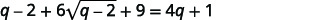方程中还有一个激进，所以我们必须重复前面的步骤。 隔离激进分子。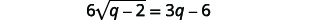两边都是正方形。 将双方分开无济于事$$6$$。 记得把$$6$$和都对齐$$\sqrt{q-2}$$。简化，然后求解新方程。分发。它是一个二次方程，所以在一边求零。将右侧考虑在内。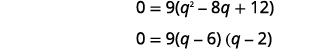使用 “零积分” 属性。支票留给你了。 解决方案是$$q=6$$和$$q=2$$。
##### 练习$$\PageIndex{19}$$

$$x=5$$

##### 练习$$\PageIndex{20}$$

$$x=0 x=4$$

## 在应用程序中使用自由基

### 对带有公式的应用程序使用问题解决策略

1. 阅读问题并确保所有文字和想法都被理解。 在适当的时候，画一个图形并用给定的信息贴上标签。
2. 确定我们在寻找什么。
3. 通过选择一个变量来表示我们要找的东西来@@ 命名它。
4. 通过编写适合情况的公式或模型将其@@ 转换为方程。 在给定的信息中替换。
5. 使用良好的代数技巧求@@ 解方程
6. 检查问题中的答案并确保答案合理。
7. 用完整的句子@@ 回答问题。

$$t=\frac{\sqrt{h}}{4}$$取的平方根$$64$$。简化分数。$$64$$英尺高度掉落的物体需要$$2$$几秒钟才能到达地面。

##### 示例$$\PageIndex{11}$$

 第 1 步：阅读问题。 第 2 步：确定我们在寻找什么。 太阳镜到达河里所花费的时间。 第 3 步：说出我们正在寻找的内容。 让 (t=\) 时间吧。 第 4 步：通过编写相应的公式将其转换为方程。 在给定的信息中替换。步骤 5：求解方程。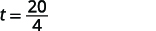第 6 步：检查问题中的答案并确保答案合理。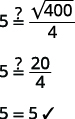$$5$$秒看起来像是合理的时间长度吗？ 是的。 步骤 7：回答方程式。 太阳镜需要$$5$$几秒钟才能到达河边。
##### 练习$$\PageIndex{21}$$

$$9$$

##### 练习$$\PageIndex{22}$$

$$3.5$$

$$s=\sqrt{24 d}$$

##### 示例$$\PageIndex{12}$$

 第 1 步：阅读问题。 第 2 步：确定我们在寻找什么。 汽车的速度。 第 3 步：说出我们要找的内容。 让速度$$s=$$加快。 第 4 步：通过编写相应的公式将其转换为方程。 在给定的信息中替换。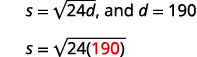步骤 5：求解方程。四舍$$1$$五入到小数位。刹车前汽车的速度为每小时$$67.5$$英里数。
##### 练习$$\PageIndex{23}$$

$$42.7$$英尺

##### 练习$$\PageIndex{24}$$

$$54.1$$英尺

• 求解涉及单个自由基的方程
• 用自由基和有理指数求解方程
• 求解激进方程
• 求解激进方程
• 激进方程应用

## 关键概念

• 二项式方块
$$\begin{array}{l}{(a+b)^{2}=a^{2}+2 a b+b^{2}} \\ {(a-b)^{2}=a^{2}-2 a b+b^{2}}\end{array}$$
• 求解激进方程
1. 在方程的一侧分离出其中一个激进项。
2. 将方程的两边提高到指数的幂次方。
3. 还有其他激进分子吗？
如果是，请再次重复步骤 1 和步骤 2。
如果不是，则求解新方程。
4. 检查原始方程式中的答案。
• 使用公式的应用程序的问题解决策略
1. 阅读问题并确保所有文字和想法都被理解。 在适当的时候，画一个图形并用给定的信息贴上标签。
2. 确定我们在寻找什么。
3. 通过选择一个变量来表示我们要找的东西来命名它。
4. 通过编写适合情况的公式或模型将其转换为方程。 在给定的信息中替换。
5. 使用良好的代数技巧求解方程。
6. 检查问题中的答案并确保答案合理。
7. 用完整的句子回答问题。
• 坠落物体
• 在地球上，如果物体从$$h$$英尺的高度掉落，则使用公式得出到达地面所需的时间（以秒为单位）$$t=\frac{\sqrt{h}}{4}$$
• 汽车的防滑痕迹和速度
• 如果防滑痕迹的长度为$$d$$英尺$$s$$，则可以使用公式得出刹车前汽车的速度$$s=\sqrt{24d}$$